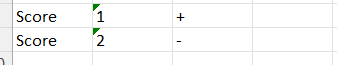# Assigning Values to choices and later summing them up

Am trying to add values to a single choice question where the option are + and -. For + I want to assign a 1 and - assign a 0. At the end of all questions I want to do a summation. Am unable to find the solution.1. Change the name of the - score to 0 (instead of 2).
2. Add a calculate type ScoreSum variable with a calculation: coalesce(number(\${I_1}), 0) + … + coalesce(number(\${I_6}), 0).

It should also work without number() and if all scores are mandatory, without coalesce( ).

You can find similar examples with the (famous) search function of this forum, e.g. Select One questions calculation.

2 Likes